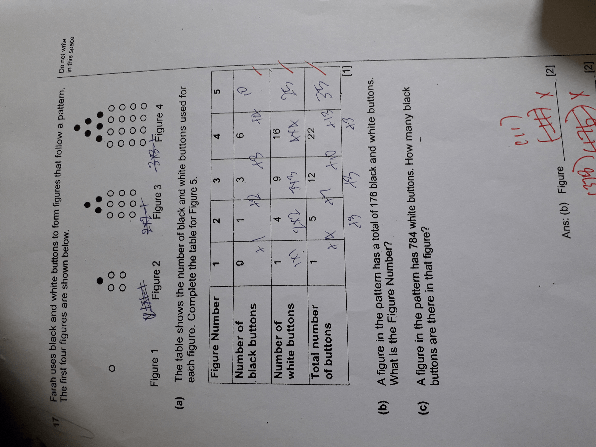# QuestionHelp me to solve (b) and (c)

With Clear working

White buttons are square of the figure number, since total dots is 176 , try 10 or 11.

b) White button = 11 x 11 = 121

Black button = (11 x 10) / 2 = 110/2 = 55

121 + 55 = 176

Pattern number  = 11

(c) sqrt ( 784) = 28

Black dots = (28 x 27) /2 = 378

0 Replies 0 Likes